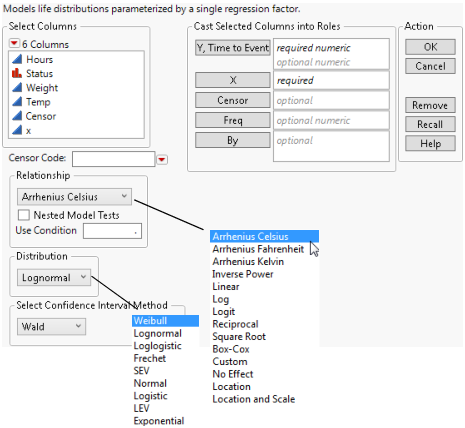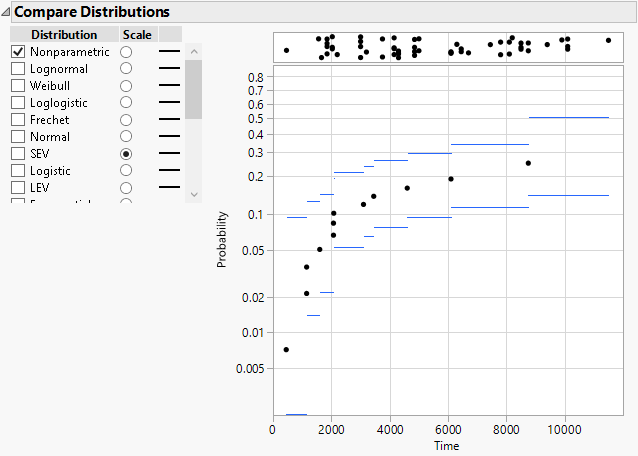Publication date: 08/13/2020

## Change the Scale

In the initial Compare Distributions report, the probability and time axes are linear. But suppose that you want to see distribution estimates on a Fréchet scale.1

1. Follow step 1 through step 5 in Example of the Life Distribution Platform.

2. In the Compare Distributions report, select Fréchet in the Scale column.

3. Click the Life Distribution red triangle and select Interval Type > Pointwise.

Figure 3.14 Nonparametric Estimates with a Fréchet Probability ScaleUsing a Fréchet scale, the nonparametric estimates approximate a straight line, meaning that a Fréchet fit might be reasonable.

4. Select SEV in the Scale column.

The nonparametric estimates no longer approximate a straight line. You now know that the SEV distribution is not appropriate.

Figure 3.15 Nonparametric Estimates with a SEV Probability ScaleUsing different scales is sometimes referred to as drawing the distribution on different types of probability paper.